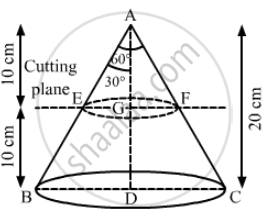Advertisement Remove all ads

# A Solid Metallic Right Circular Cone 20 Cm High and Whose Vertical Angle is 60°, is Cut into Two Parts at the Middle of Its Height by a Plane Parallel to Its - Mathematics

Sum

A solid metallic right circular cone 20 cm high and whose vertical angle is 60°, is cut into two parts at the middle of its height by a plane parallel to its base. If the frustum so obtained be drawn into a wire of diameter 1/12 cm, then find the length of the wire.

Advertisement Remove all ads

#### SolutionWe have,

Height of the metallic cone, H = 20 cm,

Height of the frustum, "h" = 20/2 = 10  "cm" and

Radius of the wire = 1/24  "cm"

Let the length of the wire be l, EG = r and BD = R

In ΔAEG,

"tan"30° = "EG"/"AG"

rArr 1/sqrt(3) = r/("H"-"h")

rArr 1/sqrt(3)="r"/(20-10)

rArr r = 10/sqrt(3) "cm"

Also, in Δ ABD,

tan 30° = "BD"/"AD"

rArr 1/sqrt(3) = "R"/"H"

rArr 1/sqrt(3) = "R"/20

rArr "R" = 20/sqrt(3)  "cm"

Now,

Volume of the wire = Volume of the frustum

rArr pi(1/24)^2l = 1/3pih (R^2 + r^2 +"Rr")

= "l"/576 = 1/3xx10xx[(20/sqrt(3))^2 + (10/sqrt(3))^2 + (20/sqrt(3))(10/sqrt(3))]

rArr "l" = 576/3xx10xx[400/3+100/3+200/3]

= "l" = 576/3xx10xx700/3

⇒ l = 448000 cm

∴ l = 4480 m

So, the length of the wire is 4480 m.

Is there an error in this question or solution?
Advertisement Remove all ads

#### APPEARS IN

RS Aggarwal Secondary School Class 10 Maths
Chapter 19 Volume and Surface Area of Solids
Exercise 19C | Q 19 | Page 912
Advertisement Remove all ads

#### Video TutorialsVIEW ALL 

Advertisement Remove all ads
Share
Notifications

View all notifications

Forgot password?
Course